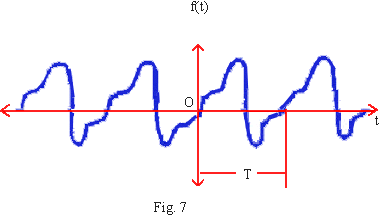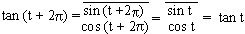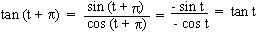Support the Monkey! Tell All your Friends and TeachersHome MonkeyNotes Printable Notes Digital Library Study Guides Study Smart Parents Tips College Planning Test Prep Fun Zone Help / FAQ How to Cite New Title Request

 5.2 Periodic Functions A periodic function can be defined as any function for which, f (t) = f (t + T)   ......(i) for all t. The smallest constant T which satisfies (i) is called the period (or the fundamental period) of the function. By iteration of (i), we have f(t) = f (t + nT), n = 0, ± 1, ± 2, ±3,    ....... (ii) An example of a periodic function is shown in fig. 7A very simple but interesting case we can observe from the unit circle. Its circumference is 2pr, but r = 1 \ C = 2p. If any point P moves around it for a distance of 2p, it returns to where it started. In other words, for any value of 't' if 2p is added or subtracted, P(t) has the same coordinates. (1)     From this discussion it follows that, sin (t +2p) = sin t sin (t - 2p) = sin t cos (t + 2p) = cos t cos (t - 2p) = cos t If 'n' is an integer sin (t + 2np) = sin t       cos (t + 2np) = cos t \ sine and cosine functions have the property as given in (ii). Therefore sine and cosine functions are 'periodic functions'. Also we note that no real number less than 2p can be made of period of sine and cosine functions. Therefore '2p' is the 'fundamental period' of sin t and cos t. (2) Let us now consider tan t, t Î R, cos t ¹ 0\ tan t is periodic and periodic with period 2p We further observe that,p is also period of tan t. Since p < 2p, the fundamental period of tan t cannot be 2p. Index
 Search: All Products Books Popular Music Classical Music Video DVD Toys & Games Electronics Software Tools & Hardware Outdoor Living Kitchen & Housewares Camera & Photo Cell Phones Keywords: